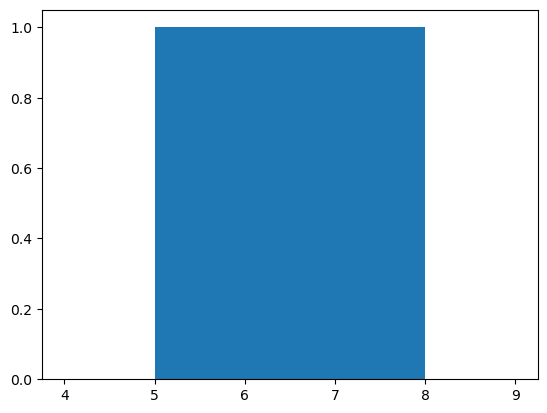# Matplotlib - Histogram

A histogram is an accurate representation of the distribution of numerical data. It is an estimate of the probability distribution of a continuous variable. It is a kind of bar graph.

To construct a histogram, follow these steps −

• Bin the range of values.
• Divide the entire range of values into a series of intervals.
• Count how many values fall into each interval.

The bins are usually specified as consecutive, non-overlapping intervals of a variable.

The matplotlib.pyplot.hist() function plots a histogram. It computes and draws the histogram of x.

### Parameters

The following table lists down the parameters for a histogram −

 x array or sequence of arrays bins integer or sequence or ‘auto’, optional optional parameters range The lower and upper range of the bins. density If True, the first element of the return tuple will be the counts normalized to form a probability density cumulative If True, then a histogram is computed where each bin gives the counts in that bin plus all bins for smaller values. histtype The type of histogram to draw. Default is ‘bar’ ‘bar’ is a traditional bar-type histogram. If multiple data are given the bars are arranged side by side. ‘barstacked’ is a bar-type histogram where multiple data are stacked on top of each other. ‘step’ generates a lineplot that is by default unfilled. ‘stepfilled’ generates a lineplot that is by default filled.

Following example plots a histogram of marks obtained by students in a class. Four bins, 0-25, 26-50, 51-75, and 76-100 are defined. The Histogram shows number of students falling in this range.

```from matplotlib import pyplot as plt
import numpy as np
fig,ax = plt.subplots(1,1)
a = np.array([22,87,5,43,56,73,55,54,11,20,51,5,79,31,27])
ax.hist(a, bins = [0,25,50,75,100])
ax.set_title("histogram of result")
ax.set_xticks([0,25,50,75,100])
ax.set_xlabel('marks')
ax.set_ylabel('no. of students')
plt.show()
```

The plot appears as shown below −